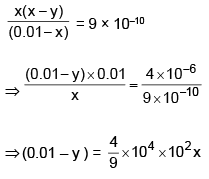Courses

# Ionic Equilibrium MCQ - 1 (Advance)

## 20 Questions MCQ Test Chemistry for JEE Advanced | Ionic Equilibrium MCQ - 1 (Advance)

Description
This mock test of Ionic Equilibrium MCQ - 1 (Advance) for JEE helps you for every JEE entrance exam. This contains 20 Multiple Choice Questions for JEE Ionic Equilibrium MCQ - 1 (Advance) (mcq) to study with solutions a complete question bank. The solved questions answers in this Ionic Equilibrium MCQ - 1 (Advance) quiz give you a good mix of easy questions and tough questions. JEE students definitely take this Ionic Equilibrium MCQ - 1 (Advance) exercise for a better result in the exam. You can find other Ionic Equilibrium MCQ - 1 (Advance) extra questions, long questions & short questions for JEE on EduRev as well by searching above.
*Multiple options can be correct
QUESTION: 1

### Which of the following statement(s) is/are correct?

Solution:

(A)
pH of 10–8 m sol. of HCl is 6.97 (consider the H+ from H2O also) (B)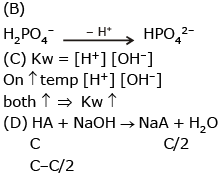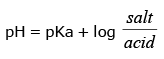*Multiple options can be correct
QUESTION: 2

### A 2.5 gm impure sample containing weak monoacidic base (Mol. wt. = 45) is dissolved in 100 ml water and titrated with 0.5 M HCl when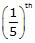of the base was neutralised the pH was found to be 9 and at equivalent point pH of solution is 4.5. Given : All data at 25º C & log 2 = 0.3. Select correct statement(s).

Solution:

BOH + HCl  → BCl + H2O
100 m 0.5 V 20 M
100 M – 0.5 V  0  = 80 M
14–a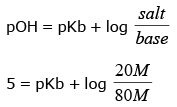⇒ pkb = 5 – log 0.25 ⇒ pkb = 5.6
⇒ Kb = 2.5 × 10–6
greater than 10–6

*Multiple options can be correct
QUESTION: 3

### Select incorrect statement(s).

Solution:

At eq. point pH = 4.5
pOH = 9.5
pOH = 1/2 [Pkb + pkw + hc ]
9.5 = 1/2 [5.6 + 14 + hc] ⇒ C = 0.25
(C) Total volume be (100 + V)
0.25 × (100 + V) = 0.5 × C  ⇒  V = 100 ml (D) 100 M = 0.5 V
100 × M = 0.5 × 100
M = 0.5
100 ml _________ 0.5 mole
100 ml _________ 0.055 mole of base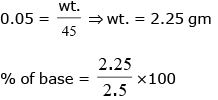90%

*Multiple options can be correct
QUESTION: 4

Which of the following is true for alkaline aqueous solution?

Solution:

For alkaline solution
pH > 7

QUESTION: 5

Ionisation constant of formic acid is 1.8 * 1.8*10−4 at 298 K. In 0.1 NHCOOH the percentage ionisation of HCOOH acid is

Solution:
QUESTION: 6

A solution contains HCl, Cl2HC COOH & CH3COOH at concentation 0.09 M in HCl, 0.09 M in Cl2HC COOH & 0.1 M in CH3COOH. pH for the solution is 1. Ionization constant of CH3COOH = 105. What is the magnitude of K for dichloroacetic acid ?

Solution: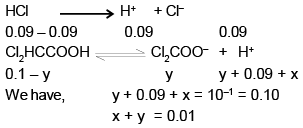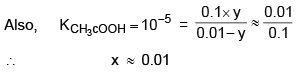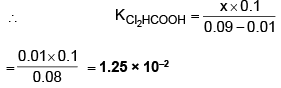QUESTION: 7

A solution of chloroacetic acid, ClCH2COOH containing 9.45 grams in 500 ml of the solution has a pH of 2.0. What is the degree of ionization the acid.

Solution:

MW of ClCH2COOH = 44.5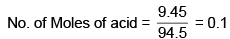∴ [acid] = 0.1 × 2 = 0.2 M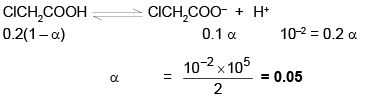QUESTION: 8

The Kw of water at two different temperature is :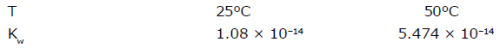Assuming that ΔH of any reaction is independent of temperature, calculate the enthalpy of neutralization of strong acid and strong base.

Solution: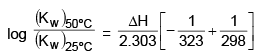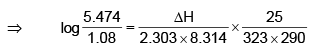(ΔH)ionization of H2O = 51.963 KJ/mol
∴ (ΔH)Neutralization  = – 51.963 KJ/mol

QUESTION: 9

The equilibrium constant of the reaction.

2Ag(s) + 2I- + 2H2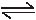2Agl(s) + H2(g) + 2OH-

is 1.2 × 10-23 at 25°C. Calculate the pH of a solution at equilibrium with the iodine ion concentation = 0.10 and the pressure of H2 gas = 0.60 atm.

Solution: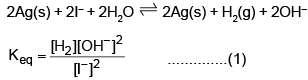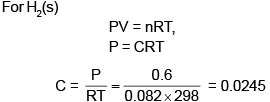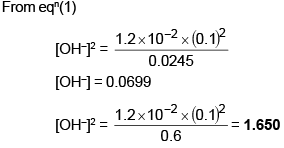QUESTION: 10

When a 40 mL of a 0.1 M weak base in titrated with 0.16 M HCl, the pH of the solution at the end point is 5.23. What will be the pH if 15 mL of 0.12 M NaOH is added to the resulting solution.

Solution: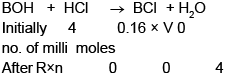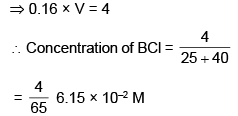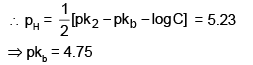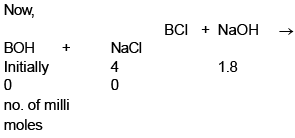The mixture is buffer of BOH and BCl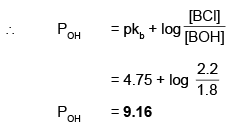QUESTION: 11

How many moles of sodium hydroxide can be added to 1.00 L of a solution 0.1 M in NH3 & 0.1 M in NH4Cl without changing the pOH by mor than 1.00 unit ? Assume no change in volume. Kb(NH3) = 1.8 × 10-5.

Solution:

NH4Cl →  NH4+ + Cl
NH3 + H2O ® NH4+ + OH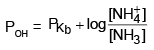= 5 –log 1.8 + log(1)
= 4.74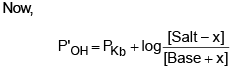where x is moles of NaOH added
⇒ P'OH -PKb = P'OH -POH = 1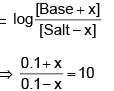⇒ 0.1 + x = 1 – 10x
⇒ 11 x = 0.9
⇒ x  = 0.0818 moles

QUESTION: 12

20 ml of a solution of 0.1 M CH3COOH solution is being titrated against 0.1 M NaOH solution. The pH vaues after the addition of 1 ml & 19 ml of NaOH are (pH)1 & (pH)2, what is ΔpH ?

Solution:

CH3COOH + NaOH → CH3COONa + H2O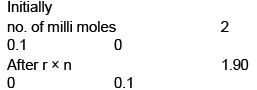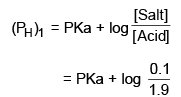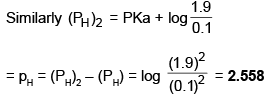QUESTION: 13

Calculate the OH- concentration and the H3PO4 concentration of a solution prepared by dissolving 0.1 mol f Na3PO4 in sufficient water to make 1 l of solution. K1 = 7.1 × 10-3, K2 = 6.3 × 10-8, K3 = 4.5 × 10-13.

Solution:

Na3PO4 → 3Na+ + PO43–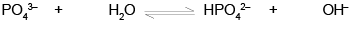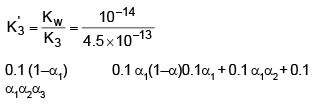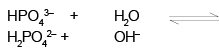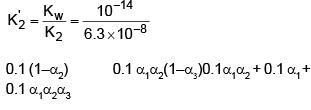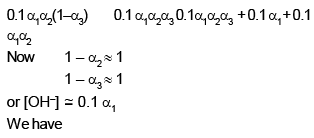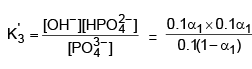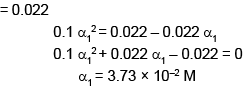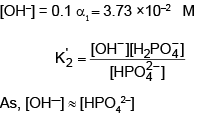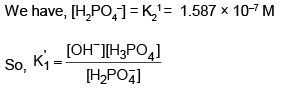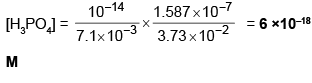QUESTION: 14

Solubility product of AgCl is 2.8 × 10-10 at 25ºC. Calculate solubility of the salt in 0.1 M AgNO3 solution -

Solution:

Ksp(AgCl) = 2.8 × 10–10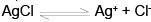2.8 × 10–10 = (s + 0.1)s
s = 2.8 × 10–9

QUESTION: 15

Equilibrium constant for the acid ionization of Fe3+ to Fe(OH)+2 and H+ is 6.5 × 10-3. What is the max. pH, which could be used so that at least 95% of the total Fe3+ in a dilute solution. exists are Fe3+.

Solution: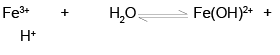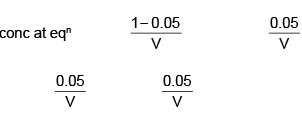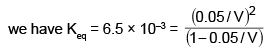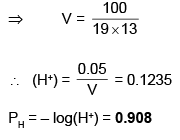QUESTION: 16

A solution of weak acid HA was titrated with base NaOH. The equivalence point was reaced when 36.12 ml of 0.1 M NaOH has been added. Now 18.06 ml of 0.1 M HCl were added to titration solution, the pH was found to be 4.92. What will be the pH of the solution obtained by mixing 10 ml 0.2 M NaOH and 10 ml of 0.2 M HA.

Solution: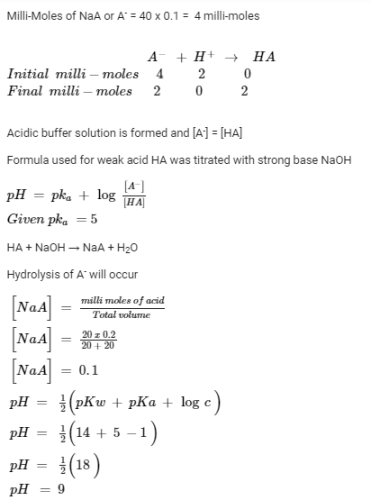QUESTION: 17

A weak base BOH was titrated against a strong acid. The pH at 1/4 the equivalence point was 9.24. Enough strong base was now added (6m eq.) to completely convert the salt. The total volume was 50 ml. Find the pH at this point.

Solution:

BOH + HCl → BCl + H2O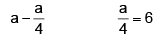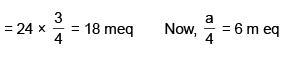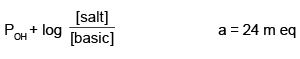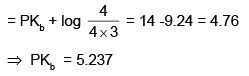Now, BCl + NaOH → BOH + NaCl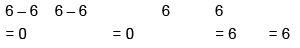Total BOH = 6 + 18 = 24 m eq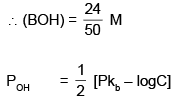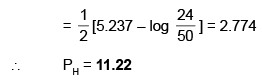QUESTION: 18

At 25°C, will a precipitate of Mg(OH)2 form in a 10-4 M solution of Mg(NO3)2 if pH of the solution is adjusted to 9.0. Ksp [Mg(OH)2] = 10-11 M3. At what min value of pH will precipitation start.

Solution: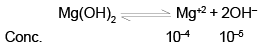As, Ionic product, 10–4 × (10–5)2 < Ksp
No ppt occurs.
Min  value of [OH–] for pptn to occur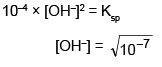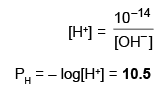QUESTION: 19

What is the solubility of AgCl in 0.20 M NH3 ?

Given : Ksp(AgCl) = 1.7 × 10-10 M2, K1 = [Ag(NH3)+] / [Ag+] [NH3] = 2.33 × 103M-1 and

K2 = [Ag(NH3)2+] / [Ag (NH3)[NH3] = 7.14 × 103 M-1

Solution:

If x be the concentration of AgCl in the solution, then [Cl] = x
From the Ksp for AgCl, we derive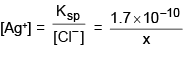If we answer that the majority of the dissolved
Ag+ goes into solution as Ag(NH3)2+ then
Ag(NH3)2+ then
Ag(NH3)2+ = x
Since two molecules of NH3 are required for every Ag(NH3)2+ ion formed, we have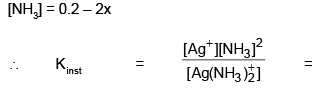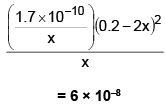∴  x = [Ag(NH3)2+] = 9.6 × 10–3 M, which is the solubility of AgCl in 0.2 M NH3

QUESTION: 20

Equal volumes of 0.02 M AgNO3 and 0.02 M HCN were mixed. Calculate [Ag+] at equilibrium. Take Ka(HCN) = 9 × 10-10, Ksp (AgCN) =4 × 10-6.

Solution: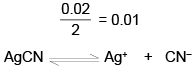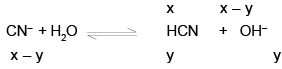W e have, Ksp(AgCN) = x (x – y) = 4 × 10–16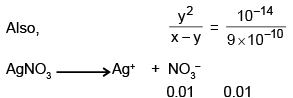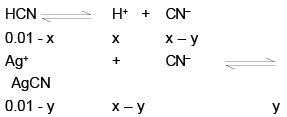(0.01-y) (x-y) = Ksp(AgCN) = 4 × 106